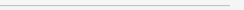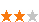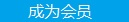您可以捐助，支持我们的公益事业。 1元 10元 50元 认证码：必填求知 文章 文库 Lib 视频 iProcess 课程 角色 咨询 工具 讲座 Modeler Code要资料订阅捐助
JavaScript 开发的 45 个经典技巧1011 次浏览     评价： 好 中 差

1 前言

JavaScript 是一种直译式脚本语言，也是一种动态类型、弱类型、基于原型的语言。它的解释器被称为 JavaScript 引擎，为浏览器的一部分，因此其也是一种被广泛用于客户端的脚本语言，最早是在 HTML 网页上使用，用来给 HTML 网页增加动态功能。现在，JavaScript 已经成为了当今使用最为广泛、最受欢迎的语言之一，多了解一些 JavaScript 的使用技巧，当然是有益无害啦！

2 内容

JavaScript 是一个绝冠全球的编程语言，可用于 Web 开发、移动应用开发（PhoneGap 和 Appcelerator）、服务器端开发（Node.js 和 Wakanda）等等。JavaScript 还是很多新手踏入编程世界的第一个语言，既可以用来显示浏览器中的简单提示框，也可以通过 nodebot 或 nodruino 来控制机器人。能够编写结构清晰、性能高效的 JavaScript 代码的开发人员，现如今已成了招聘市场最受追捧的人。

1. 首次为变量赋值时务必使用 var 关键字

2. 使用 === 取代 ==

==和!=操作符会在需要的情况下自动转换数据类型。但===和!==不会，它们会同时比较值和数据类型，这也使得它们要比==和!=快。

 ` === 10 // is false == 10 // is true'10' == 10 // is true'10' === 10 // is false [] == 0 // is true [] === 0 // is false '' == false // is true but true == "a" is false '' === false // is false`

3. underfined、null、0、false、NaN、空字符串的逻辑结果均为 false

4. 行尾使用分号

5. 使用对象构造器

 `function Person(firstName, lastName){ this.firstName = firstName; this.lastName = lastName;}var Saad = new Person("Saad", "Mousliki");`

6. 小心使用 typeof、instanceof 和 contructor

typeof：JavaScript 一元操作符，用于以字符串的形式返回变量的原始类型，注意，typeof null 也会返回 object，大多数的对象类型（数组 Array、时间 Date 等）也会返回 object；

instanceof：JavaScript操作符，会在原型链中的构造器中搜索，找到则返回true，否则返回false；

contructor：内部原型属性，可以通过代码重写。

 `var arr = ["a", "b", "c"];typeof arr; // 返回 "object" arr instanceof Array // truearr.constructor(); //[]`

7. 使用自调用函数

 `(function(){ // 置于此处的代码将自动执行})(); (function(a, b){ var result = a + b; return result;})(10, 20)`

8. 从数组中随机获取成员

 `var items = [12, 548 , 'a' , 2 , 5478 , 'foo' , 8852, , 'Doe' , 2145 , 119];var randomItem = items[Math.floor(Math.random() * items.length)];`

9. 获取指定范围内的随机数

 `var x = Math.floor(Math.random() * (max - min + 1)) + min;`

10. 生成从 0 到指定值的数字数组

 `var numbersArray = [] , max = 100;for( var i=1; numbersArray.push(i++) < max;); // numbers = [1,2,3 ... 100]`

11. 生成随机的字母数字字符串

 `function generateRandomAlphaNum(len) { var rdmString = ""; for( ; rdmString.length < len; rdmString += Math.random().toString(36).substr(2)); return rdmString.substr(0, len);}`

12. 打乱数字数组的顺序

 `var numbers = [5, 458 , 120 , -215 , 228 , 400 , 122205, -85411];numbers = numbers.sort(function(){ return Math.random() - 0.5});/* numbers 数组将类似于 [120, 5, 228, -215, 400, 458, -85411, 122205] */`

13. 字符串去空格

Java、C# 和 PHP 等语言都实现了专门的字符串去空格函数，但 JavaScript 中是没有的，可以通过下面的代码来为 String 对象定义一个trim函数：

 `String.prototype.trim = function(){return this.replace(/^\s+|\s+\$/g, "");};`

14. 数组之间追加

 `var array1 = [12 , "foo" , {name "Joe"} , -2458];var array2 = ["Doe" , 555 , 100];Array.prototype.push.apply(array1, array2);/* array1 值为 [12 , "foo" , {name "Joe"} , -2458 , "Doe" , 555 , 100] */`

15. 对象转换为数组

 `var argArray = Array.prototype.slice.call(arguments);`

16. 验证是否是数字

 `function isNumber(n){ return !isNaN(parseFloat(n)) && isFinite(n);}`

17. 验证是否是数组

 `function isArray(obj){ return Object.prototype.toString.call(obj) === '[object Array]' ;}`

 `Array.isArray(obj); // its a new Array method`

 `var myFrame = document.createElement('iframe');document.body.appendChild(myFrame);var myArray = window.frames[window.frames.length-1].Array;var arr = new myArray(a,b,10); // [a,b,10] // myArray 的构造器已经丢失，instanceof 的结果将不正常// 构造器是不能跨 frame 共享的arr instanceof Array; // false`

18. 获取数组中的最大值和最小值

 `var numbers = [5, 458 , 120 , -215 , 228 , 400 , 122205, -85411]; var maxInNumbers = Math.max.apply(Math, numbers); var minInNumbers = Math.min.apply(Math, numbers);`

19. 清空数组

 `var myArray = [12 , 222 , 1000 ]; myArray.length = 0; // myArray will be equal to []`

20. 不要直接从数组中 delete 或 remove 元素

 `var items = [12, 548 ,'a' , 2 , 5478 , 'foo' , 8852, , 'Doe' ,2154 , 119 ]; items.length; // return 11 delete items; // return true items.length; // return 11 /* items 结果为 [12, 548, "a", undefined × 1, 5478, "foo", 8852, undefined × 1, "Doe", 2154, 119] */`

 `var items = [12, 548 ,'a' , 2 , 5478 , 'foo' , 8852, , 'Doe' ,2154 , 119 ]; items.length; // return 11 items.splice(3,1) ; items.length; // return 10 /* items 结果为 [12, 548, "a", 5478, "foo", 8852, undefined × 1, "Doe", 2154, 119]`

21. 使用length属性截断数组

 `var myArray = [12 , 222 , 1000 , 124 , 98 , 10 ]; myArray.length = 4; // myArray will be equal to [12 , 222 , 1000 , 124]`

 `myArray.length = 10; // the new array length is 10 myArray[myArray.length - 1] ; // undefined`

22. 在条件中使用逻辑与或

 `var foo = 10; foo == 10 && doSomething(); // is the same thing as if (foo == 10) doSomething(); foo == 5 || doSomething(); // is the same thing as if (foo != 5) doSomething();`

 `function doSomething(arg1){ arg1 = arg1 || 10; // arg1 will have 10 as a default value if it’s not already set}`

23. 使用 map() 函数方法对数据循环

 `var squares = [1,2,3,4].map(function (val) { return val * val; }); // squares will be equal to [1, 4, 9, 16]`

24. 保留指定小数位数

 `var num =2.443242342;num = num.toFixed(4); // num will be equal to 2.4432`

25. 浮点计算的问题

 `0.1 + 0.2 === 0.3 // is false 9007199254740992 + 1 // is equal to 90071992547409929007199254740992 + 2 // is equal to 9007199254740994`

26. 通过 for in 循环检查对象的属性

 `for (var name in object) { if (object.hasOwnProperty(name)) { // do something with name } }`

27. 逗号操作符

 `var a = 0; var b = ( a++, 99 ); console.log(a); // a will be equal to 1 console.log(b); // b is equal to 99`

28. 临时存储用于计算和查询的变量

 `var navright = document.querySelector('#right'); var navleft = document.querySelector('#left'); var navup = document.querySelector('#up'); var navdown = document.querySelector('#down');`

29. 提前检查传入 isFinite() 的参数

 `isFinite(0/0) ; // falseisFinite("foo"); // falseisFinite("10"); // trueisFinite(10); // trueisFinite(undefined); // falseisFinite(); // falseisFinite(null); // true，这点当特别注意`

30. 避免在数组中使用负数做索引

 `var numbersArray = [1,2,3,4,5];var from = numbersArray.indexOf("foo") ; // from is equal to -1numbersArray.splice(from,2); // will return `

31. 用 JSON 来序列化与反序列化

 `var person = {name :'Saad', age : 26, department : {ID : 15, name : "R&D"} };var stringFromPerson = JSON.stringify(person);/* stringFromPerson 结果为 "{"name":"Saad","age":26,"department":{"ID":15,"name":"R&D"}}" */var personFromString = JSON.parse(stringFromPerson);/* personFromString 的值与 person 对象相同 */`

32. 不要使用 eval() 或者函数构造器

eval()和函数构造器（Function consturctor）的开销较大，每次调用，JavaScript引 擎都要将源代码转换为可执行的代码。

 `var func1 = new Function(functionCode);var func2 = eval(functionCode);`

33. 避免使用 with()

34. 不要对数组使用 for in

 `var sum = 0; for (var i in arrayNumbers) { sum += arrayNumbers[i]; }`

 `var sum = 0; for (var i = 0, len = arrayNumbers.length; i < len; i++) { sum += arrayNumbers[i]; }`

 `for (var i = 0; i < arrayNumbers.length; i++)`

35. 传给 setInterval() 和 setTimeout() 时使用函数而不是字符串

 `setInterval('doSomethingPeriodically()', 1000); setTimeout('doSomethingAfterFiveSeconds()', 5000);`

 `setInterval(doSomethingPeriodically, 1000); setTimeout(doSomethingAfterFiveSeconds, 5000);`

36. 使用 switch case 代替一大叠的 if else

37. 在 switch case 中使用数字区间

 `function getCategory(age) { var category = ""; switch (true) { case isNaN(age): category = "not an age"; break; case (age >= 50): category = "Old"; break; case (age <= 20): category = "Baby"; break; default: category = "Young"; break; }; return category; } getCategory(5); // 将返回 "Baby"`

38. 使用对象作为对象的原型

 `function clone(object) { function OneShotConstructor(){}; OneShotConstructor.prototype = object; return new OneShotConstructor(); } clone(Array).prototype ; // []`

39. HTML 字段转换函数

 `function escapeHTML(text) { var replacements= {"<": "<", ">": ">","&": "&", "\"": """}; return text.replace(/[<>&"]/g, function(character) { return replacements[character]; }); }`

40 不要在循环内部使用 try catch finally

try catch finally 中 catch 部分在执行时会将异常赋给一个变量，这个变量会被构建成一个运行时作用域内的新的变量。

 `var object = ['foo', 'bar'], i; for (i = 0, len = object.length; i

 `var object = ['foo', 'bar'], i; try { for (i = 0, len = object.length; i

41. 使用 XMLHttpRequests 时注意设置超时

XMLHttpRequests 在执行时，当长时间没有响应（如出现网络问题等）时，应该中止掉连接，可以通过setTimeout()来完成这个工作：

 `var xhr = new XMLHttpRequest (); xhr.onreadystatechange = function () { if (this.readyState == 4) { clearTimeout(timeout); // do something with response data } } var timeout = setTimeout( function () { xhr.abort(); // call error callback }, 60*1000 /* timeout after a minute */ ); xhr.open('GET', url, true); xhr.send();`

42. 处理 WebSocket 的超时

 `var timerID = 0; function keepAlive() { var timeout = 15000; if (webSocket.readyState == webSocket.OPEN) { webSocket.send(''); } timerId = setTimeout(keepAlive, timeout); } function cancelKeepAlive() { if (timerId) { cancelTimeout(timerId); } }`

keepAlive()函数可以放在 WebSocket 连接的onOpen()方法的最后面，cancelKeepAlive()放在onClose()方法的最末尾。

43. 时间注意原始操作符比函数调用快，使用 VanillaJS

 `var min = Math.min(a,b); A.push(v);`

 `var min = a < b ? a : b; A[A.length] = v;`

44. 开发时注意代码结构，上线前检查并压缩 JavaScript 代码

45. JavaScript 博大精深，这里有些不错的学习资源

Code Academy 资源：http://www.codecademy.com/tracks/javascript

Marjin Haverbekex 编写的 Eloquent JavaScript：http://eloquentjavascript.net/

John Resig 编写的 Advanced JavaScript：http://ejohn.org/apps/learn/1011 次浏览  评价: 好 中 差订阅捐助

 深度解析：清理烂代码 如何编写出拥抱变化的代码 重构-使代码更简洁优美 团队项目开发"编码规范"系列文章
 重构-改善既有代码的设计 软件重构v2 代码整洁之道 高质量编程规范
 基于HTML5客户端、Web端的应用开发 HTML 5+CSS 开发 嵌入式C高质量编程 C++高级编程

 Android手机开发（一） 理解Javascript 非典型ajax实践 彻底的Ajax javascript 使用Cookies 使用 jQuery 简化 Ajax 开发

 Struts+Spring+Hibernate 基于J2EE的Web 2.0应用开发 J2EE设计模式和性能调优 Java EE 5企业级架构设计 Java单元测试方法与技术 Java编程方法与技术

 某航空公司IT部 JavaScript实践 某电视软件 HTML5和JavaScript 中航信 JavaScript高级应用开发 大庆油田 web界面Ajax开发技术 和利时 使用AJAX进行WEB应用开发每天2个文档/视频 扫描微信二维码订阅
 订阅技术月刊 获得每月300个技术资源